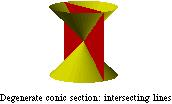index: click on a letter A B C D E F G H I J K L M N O P Q R S T U V W X Y Z A to Z index index: subject areas numbers & symbols sets, logic, proofs geometry algebra trigonometry advanced algebra & pre-calculus calculus advanced topics probability & statistics real world applications multimedia entrieswww.mathwords.com about mathwords website feedback

 Degenerate Conic Sections Plane figures that can be obtained by the intersection of a double cone with a plane passing through the apex. These include a point, a line, and intersecting lines. Like other conic sections, all degenerate conic sections have equations of the form Ax2 + Bxy + Cy2 + Dx + Ey + F = 0.See also